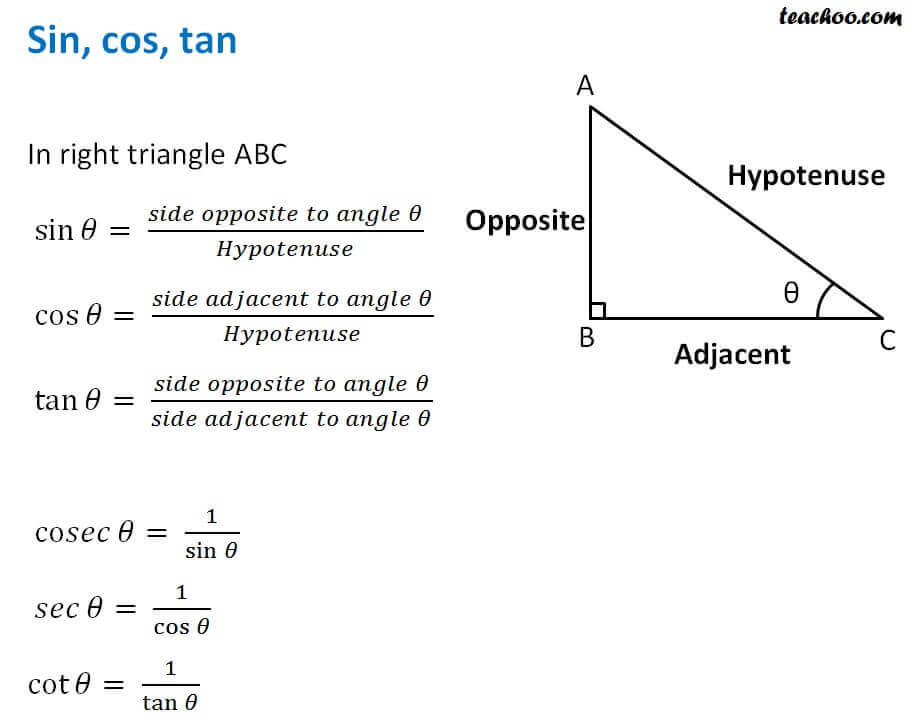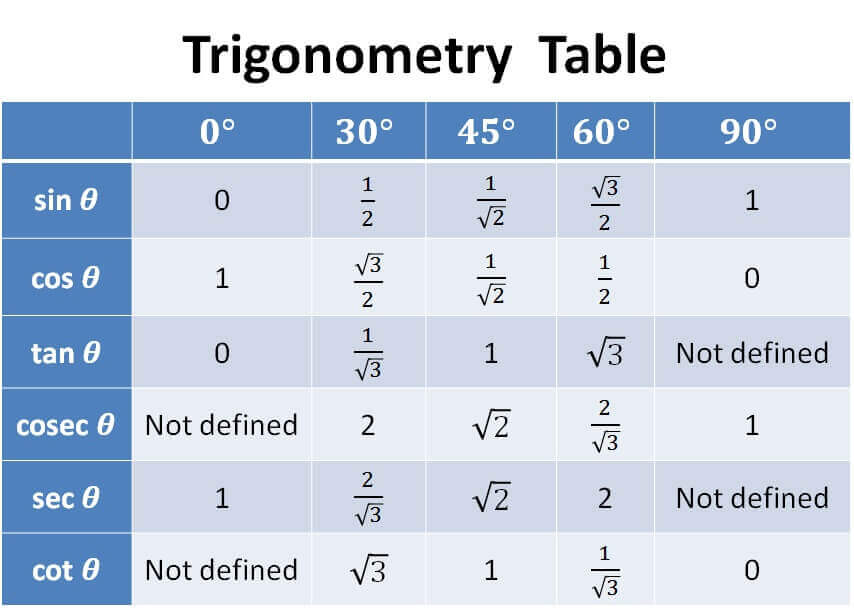## Sin, cos, tan## Value of sin, cos, tan at different angles## Basic Identities

cos 2  θ + sin 2  θ = 1

1 + tan 2  θ = sec 2  θ

1 + cot 2  θ = cosec 2  θ

Subscribe to our Youtube Channel - https://you.tube/teachoo

1. Chapter 3 Class 11 Trigonometric Functions
2. Concept wise
3. Finding Value of trignometric functions, given other functions

Transcript

In right triangle ABC sin⁡𝜃= (𝑠𝑖𝑑𝑒 𝑜𝑝𝑝𝑜𝑠𝑖𝑡𝑒 𝑡𝑜 𝑎𝑛𝑔𝑙𝑒 𝜃)/𝐻𝑦𝑝𝑜𝑡𝑒𝑛𝑢𝑠𝑒 cos⁡𝜃= (𝑠𝑖𝑑𝑒 𝑎𝑑𝑗𝑎𝑐𝑒𝑛𝑡 𝑡𝑜 𝑎𝑛𝑔𝑙𝑒 𝜃)/𝐻𝑦𝑝𝑜𝑡𝑒𝑛𝑢𝑠𝑒 tan⁡𝜃= (𝑠𝑖𝑑𝑒 𝑜𝑝𝑝𝑜𝑠𝑖𝑡𝑒 𝑡𝑜 𝑎𝑛𝑔𝑙𝑒 𝜃)/(𝑠𝑖𝑑𝑒 𝑎𝑑𝑗𝑎𝑐𝑒𝑛𝑡 𝑡𝑜 𝑎𝑛𝑔𝑙𝑒 𝜃) co𝑠𝑒𝑐⁡𝜃= 1/sin⁡𝜃 𝑠𝑒𝑐⁡𝜃= 1/cos⁡𝜃 cot⁡𝜃= 1/tan⁡𝜃

Finding Value of trignometric functions, given other functions